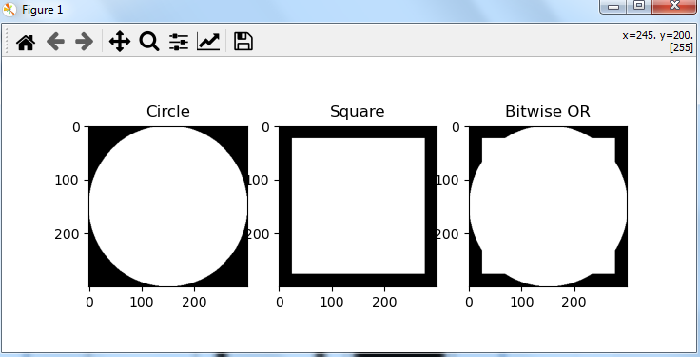# How to perform bitwise OR operation on two images in OpenCV Python?

In OpenCV, a color (RGB) image is represented as a 3-dimensional numpy array. The pixel values of an image are stored using 8 bit unsigned integers (uint8) in range from 0 to 255. The bitwise OR operation on two images is performed on the binary representation of these pixel values of corresponding images.

## Syntax

Here is the syntax to perform bitwise OR operation on two images −

cv2.bitwise_or(img1, img2, mask=None)


img1 and img2 are the two input images and mask is a mask operation.

## Steps

To compute bitwise OR between two images, you can use the steps given below −

Import the required libraries OpenCV, Numpy and Matplotlib. Make sure you have already installed them.

import cv2
import numpy as np
import matplotlib as plt


Read the images using cv2.imread() method. The width and height of images must be the same.

img1 = cv2.imread('waterfall.jpg')


Compute the bitwise OR on two images using cv2.biwise_or(img1, img2).

or_img = cv2.bitwise_or(img1,img2)


Display the bitwise OR image

cv2.imshow('Bitwise OR Image', or_img)
cv2.waitKey(0)
cv2.destroyAllWindows()


We will use the following images as the Input Files in the examples below.## Example 1

In the Python program below, we compute the bitwise OR on the two color images.

# import required libraries
import cv2

# read two images. The size of both images must be the same.

# compute bitwise OR on both images
or_img = cv2.bitwise_or(img1,img2)

# display the computed bitwise OR image
cv2.imshow('Bitwise OR Image', or_img)
cv2.waitKey(0)
cv2.destroyAllWindows()


## Output

When you run this Python program, it will produce the following output −## Example 2

This Python program shows the application of bitwise OR operation on two images. We created two images, first a circle and second a square of the same size.

# import required libraries
import cv2
import numpy as np
import matplotlib.pyplot as plt

# define first image as a circle
img1 = np.zeros((300, 300), dtype = "uint8")
img1 = cv2.circle(img1, (150, 150), 150, 255, -1)

# define second image as a square
img2 = np.zeros((300,300), dtype="uint8")
img2 = cv2.rectangle(img2, (25, 25), (275, 275), 255, -1)

# perform bitwise OR on img1 and img2
or_img = cv2.bitwise_or(img1,img2)

# Display the bitwise OR output image
plt.subplot(131), plt.imshow(img1, 'gray'), plt.title("Circle")
plt.subplot(132), plt.imshow(img2,'gray'), plt.title("Square")
plt.subplot(133), plt.imshow(or_img, 'gray'), plt.title("Bitwise OR")

plt.show()


## Output

When you run this Python program, it will produce the following output −